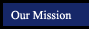CAHSEE Prep - Tutorial sources by Topic - Number Sense
 Scientific notation Scientific Notation lesson - ETAP.org ETAP Pretest Scientific Notation lesson - RegentsPrep.org ETAP Posttest Rational numbers Rational Numbers - ETAP.org ETAP Pretest Rational Numbers - RegentsPrep.org ETAP Posttest Convert fractions to decimals Converting Fractions to Decimals - ETAP.org ETAP Pretest Converting Fractions to Decimals - Learningwave.com ETAP Posttest Percentage calculations Percentage Calculations - RegentsPrep.org Solve problems involving discounts, markups, commissions Word problems with discounts/markups/commissions - PurpleMath.com Multiply and divide expressions involving exponents Multiply and divide exponential expressions - RegentsPrep.org Multiply, divide and simplify rational numbers Multiply/Divide/Simplify Rational Numbers - RegentsPrep.org Raising to a power and square roots Raising to a Power/Square Roots - RegentsPrep.org Absolute Value Absolute Value - RegentsPrep.org

Online Scientific Calculator from Calculator.com

Math Glossary
(more sophisticated than it looks!)